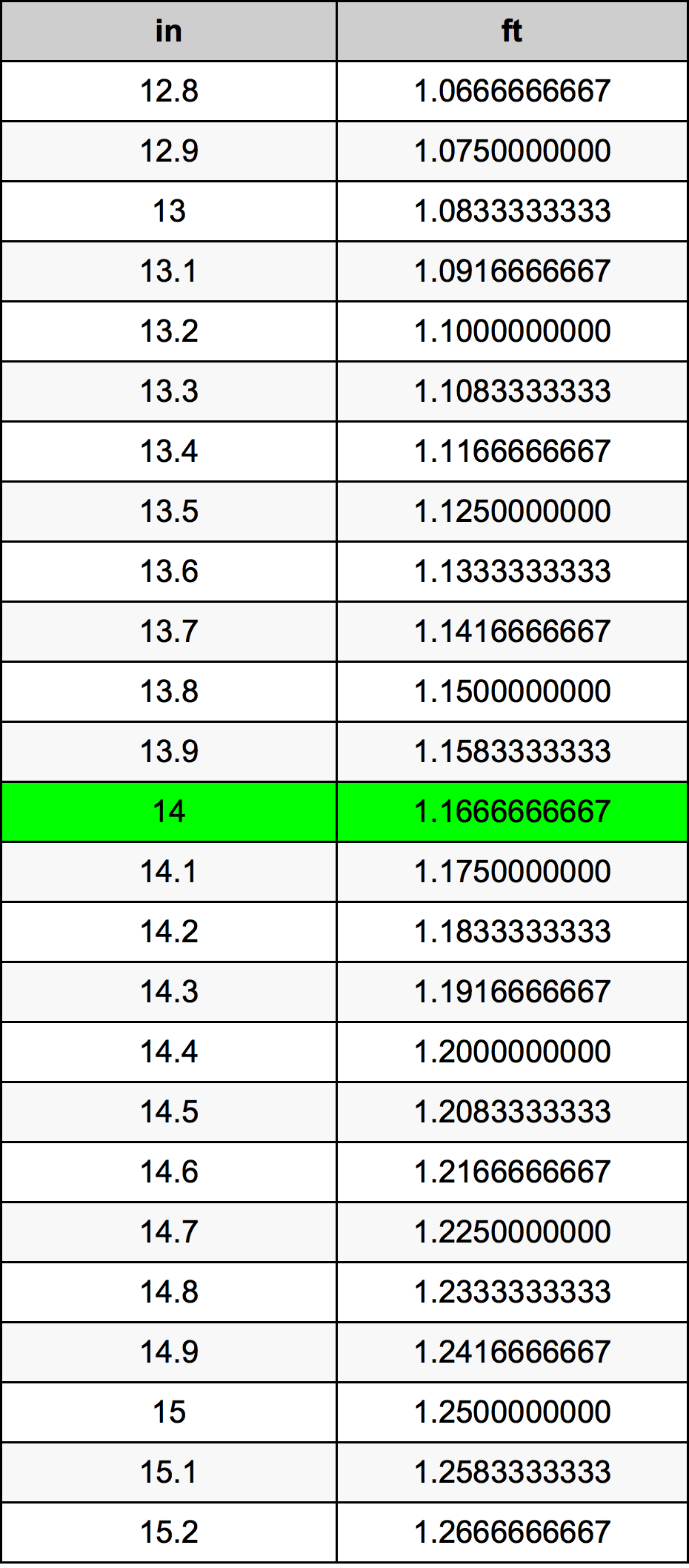Inches To Feet

# 14 in to ft14 Inches to Feet

in
=
ft

## How to convert 14 inches to feet?

 14 in * 0.0833333333 ft = 1.1666666667 ft 1 in
A common question is How many inch in 14 foot? And the answer is 168.0 in in 14 ft. Likewise the question how many foot in 14 inch has the answer of 1.1666666667 ft in 14 in.

## How much are 14 inches in feet?

14 inches equal 1.1666666667 feet (14in = 1.1666666667ft). Converting 14 in to ft is easy. Simply use our calculator above, or apply the formula to change the length 14 in to ft.

## Convert 14 in to common lengths

UnitUnit of length
Nanometer355600000.0 nm
Micrometer355600.0 µm
Millimeter355.6 mm
Centimeter35.56 cm
Inch14.0 in
Foot1.1666666667 ft
Yard0.3888888889 yd
Meter0.3556 m
Kilometer0.0003556 km
Mile0.0002209596 mi
Nautical mile0.0001920086 nmi

## What is 14 inches in ft?

To convert 14 in to ft multiply the length in inches by 0.0833333333. The 14 in in ft formula is [ft] = 14 * 0.0833333333. Thus, for 14 inches in foot we get 1.1666666667 ft.

## 14 Inch Conversion Table## Alternative spelling

14 Inch to Feet, 14 Inch in Feet, 14 Inches to Foot, 14 Inches in Foot, 14 Inches to ft, 14 Inches in ft, 14 in to ft, 14 in in ft, 14 Inch to Foot, 14 Inch in Foot, 14 Inches to Feet, 14 Inches in Feet, 14 in to Foot, 14 in in Foot